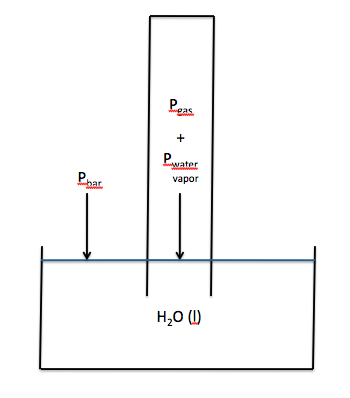# Question da319

Apr 5, 2017

The partial pressure of the gas collected is 204.16 kPa.

#### Explanation:Since the gas must bubble through the water before being collected, it becomes saturated with water vapour.

When the water levels inside and outside the container are equal, the $\text{pressure of the gas + the pressure of the water vapour = the barometric pressure}$

The vapour pressure ${p}_{\textrm{v a p}}$ of water at 25 °C is 3.1690 kPa.

p_text(gas) = p_text(bar) - p_text(vap) = "207.33 kPa - 3.1690 kPa" = "204.16 kPa"#